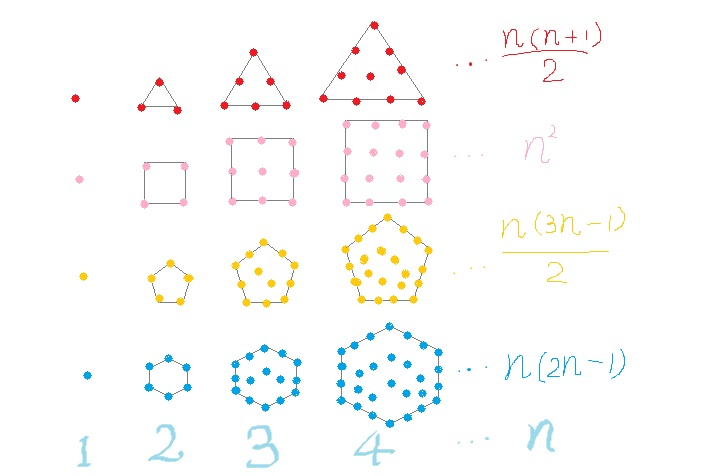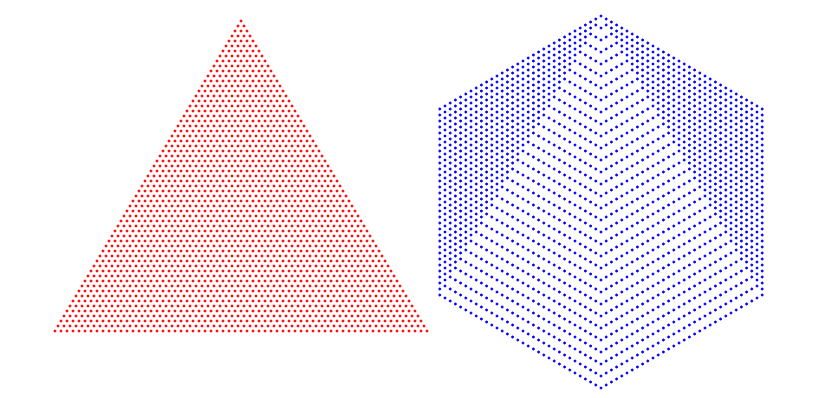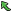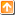## 7555: 2016Time Limit(Common/Java):2000MS/6000MS     Memory Limit:65536KByte
Total Submit: 15            Accepted:6

Description

In mathematics, a polygonal number is a number represented as dots or pebbles arranged in the shape of a regular polygon. The dots are thought of as alphas (units). These are one type of 2-dimensional figurate numbers. The following picture shows how triangular numbers, square numbers, pentagonal numbers and hexagonal numbers represented as dots arranged in the shape of corresponding regular polygon.2016 is not only a leap year but also a triangular and hexagonal year. If you are patient enough, you can count the number of the dots in the left triangle or in the right hexagon in the following picture. The number of dots in each shape is 2016.Therefore, 2016 is a triangular-hexagonal-leap year. The previous triangular-hexagonal-leap year is 1540 and the next is 2556. So living to see 2016 is very rare experience.

You task is to list the triangular-hexagonal-leap years from 2016 to 990528. 990528 is also a triangular-hexagonal-leap year.

Input

This problem has no input.

Output

Please print each triangular-hexagonal-leap year in increasing order.

For example, if you are asked to list the triangular-hexagonal-leap years from 780 to 2556, the output should be:

780
1128
1540
2016
2556

Sample Input

Sample Output

Source

ZOJ

|Back |   |Top|